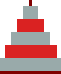## Er's TH algorithm can be made fasterM. Kolar
December 3-6, 2000

What slows down the Er's TH algorithm on some platforms is the use of the remainder operator %. Er uses % for cyclic rotations of three integers 1,2,3 right or left. The left cyclic rotation replaces j=1,2,3 by l=3,1,2, and the right cyclic rotation j=1,2,3 by r=2,3,1. The original Er's code uses the following expressions:

```	l = (j-1)%3
r = (j+1)%3
```
These can be replaced by bit operations which are faster (much faster for some compilers):
```	l = ~j>>(j&1)&3
r = ++j>>(j>>2<<1)
```
One can come up with other expressions giving identical results, such as:
```	r = ++j&3|j>>2
r = j+1&3|j-1>>1
l = (~j>>1&3)-(~j&1)
l = j-1|(j+6&6)>>1
```
But these are mostly more complex (and thus slower). Can you come up with something simpler?

Using these replacements, one gets the following modified Er's code:

 ```/* A faster variant of the Er's LLHanoi TH loopless algorithm with the remainder operator (%) replaced by faster bit operations performing left and right cyclic rotations of integers 1,2,3 */ #include #include #define PR (void)printf( #define PE (void)fprintf(stderr, #define ALLO(x) { if((x = (int *)malloc((n+2) * sizeof(int))) == NULL) {\ PE #x " allocation failed!\n"); exit(1); } } main(int argc, char *argv[]) /*========================*/ { int i, dir, *D, *s, n, j; n = atoi(argv); j = n+1; ALLO(D) ALLO(s) for(i=0; i<=j; i++) { D[i] = 1; s[i] = i+1; } dir = n&1; for(;;) { i = s; if(i>n) break; j=D[i]; /* PR"move disc %d from %d ", i, j); PR"to %d\n", D[i]=(i&1)==dir ? ~j>>(j&1)&3 : ++j>>(j>>2<<1)); */ D[i]=(i&1)==dir ? ~j>>(j&1)&3 : ++j>>(j>>2<<1); s = 1; s[i-1] = s[i]; s[i] = i+1; } } ```

This code can be found in file ErB.c (Bit-based Er) in this zip archive. The original Er's code is in file Er.c. My four algorithms are in files algo1.c through algo4.c, etc. Files hBits*.c contain two variants of an algorithm based solely on the bits of the move number.
AlgorithmDEC Alpha
UNIX 300 MHz
Cygwin on NT, Pentium II MMX 266 MHz; gcc
DLL 1.1.6B20.1B20.1/M.Khan
Er.c73.745.038.839.8
ErB.c42.530.730.631.4
algo2.c39.635.640.440.1
algo3.c37.240.541.040.8
algo4.c30.033.937.437.5
hBits00.c5.44.164.146.18
hBits0.c1.34.134.136.19
hBits.c26.818.918.622.3
hBitsVis.c85.962.673.973.0
DEC: UNIX OSF1 V 4.0; DEC C compiler (cc -O).
Cygwin (all on the same MS Windows NT 4.0 computer; gcc -s -O):
- DLL 1.1.6 is the most latest release;
- B20.1 is just over a year old;
- in the last column, Cygwin B20.1's gcc was replaced by
Mumit Khan's egcs-1.1.1 gcc compiler.

This seems to be more about testing the performance of C compilers than how fast the above TH algorithms are!